# directlabels - lineplot - example - ridge

```## complicated ridge regression lineplot ex. fig 3.8 from Elements of
## Statistical Learning, Hastie et al.
myridge <- function(f,data,lambda=c(exp(-seq(-15,15,l=200)),0)){
require(MASS)
require(reshape2)
fit <- lm.ridge(f,data,lambda=lambda)
X <- data[-which(names(data)==as.character(f[]))]
Xs <- svd(scale(X)) ## my d's should come from the scaled matrix
dsq <- Xs\$d^2
## make the x axis degrees of freedom
df <- sapply(lambda,function(l)sum(dsq/(dsq+l)))
D <- data.frame(t(fit\$coef),lambda,df) # scaled coefs
molt <- melt(D,id=c("lambda","df"))
## add in the points for df=0
limpts <- transform(subset(molt,lambda==0),lambda=Inf,df=0,value=0)
rbind(limpts,molt)
}
data(prostate,package="ElemStatLearn")
pros <- subset(prostate,train==TRUE,select=-train)
m <- myridge(lpsa~.,pros)
library(lattice)
p <- xyplot(value~df,m,groups=variable,type="o",pch="+",
panel=function(...){
panel.xyplot(...)
panel.abline(h=0)
panel.abline(v=5,col="grey")
},
xlim=c(-1,9),
main="Ridge regression shrinks least squares coefficients",
ylab="scaled coefficients",
sub="grey line shows coefficients chosen by cross-validation",
xlab=expression(df(lambda)))
```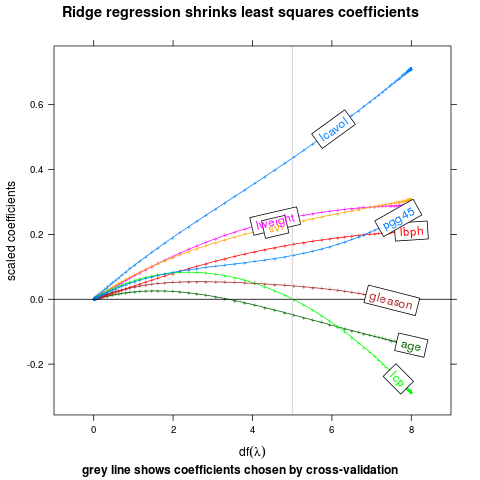Draw a box with the label inside, at the point furthest away from the plot border and any other curve. ```direct.label(p,"angled.boxes") ```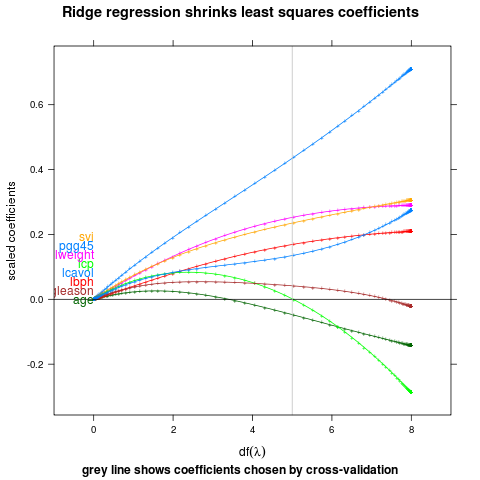Label first points, bumping labels up if they collide. ```direct.label(p,"first.bumpup") ```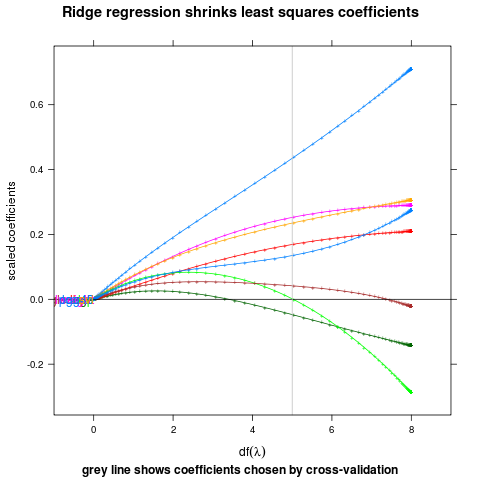Positioning Method for the first of a group of points. ```direct.label(p,"first.points") ```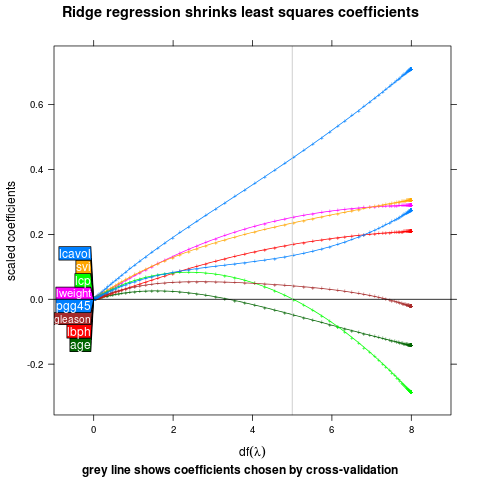Draw a speech polygon to the first point. ```direct.label(p,"first.polygons") ```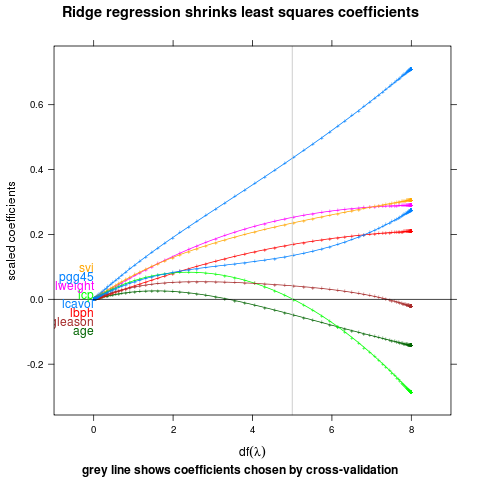Label first points from QP solver that ensures labels do not collide. ```direct.label(p,"first.qp") ```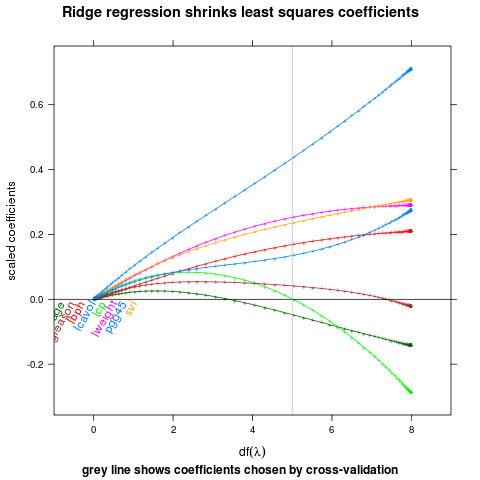Label points at the zero before the first nonzero y value. ```direct.label(p,"lasso.labels") ```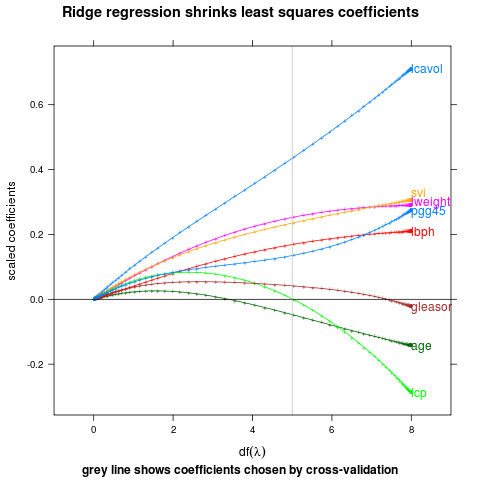Label last points, bumping labels up if they collide. ```direct.label(p,"last.bumpup") ```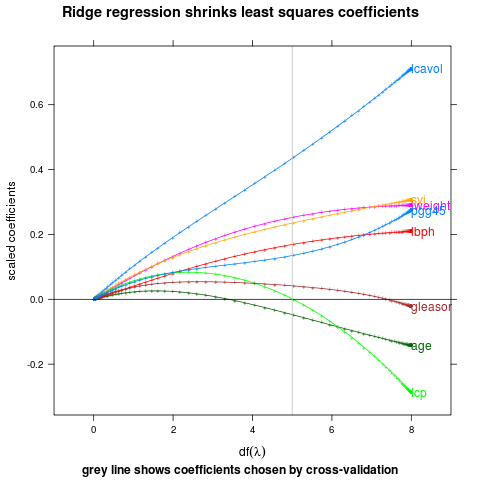Positioning Method for the last of a group of points. ```direct.label(p,"last.points") ```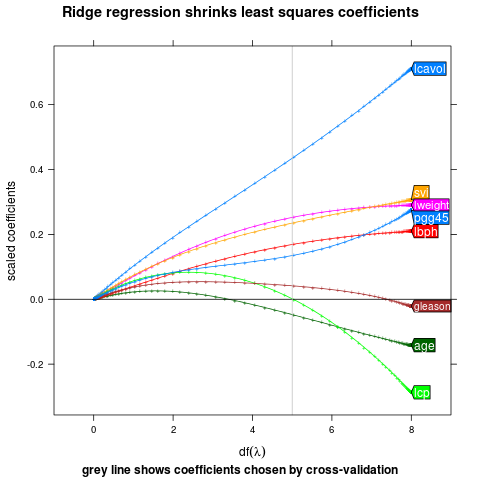Draw a speech polygon to the last point. ```direct.label(p,"last.polygons") ```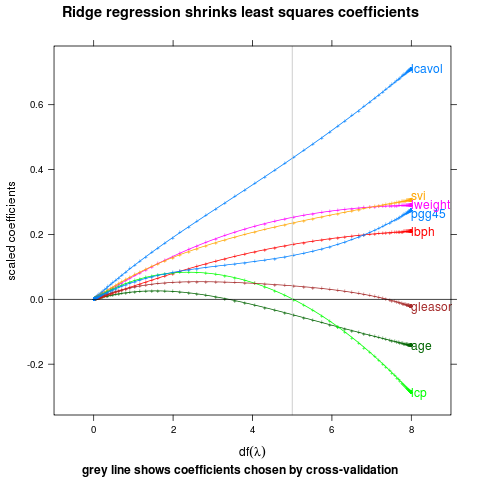Label last points from QP solver that ensures labels do not collide. ```direct.label(p,"last.qp") ```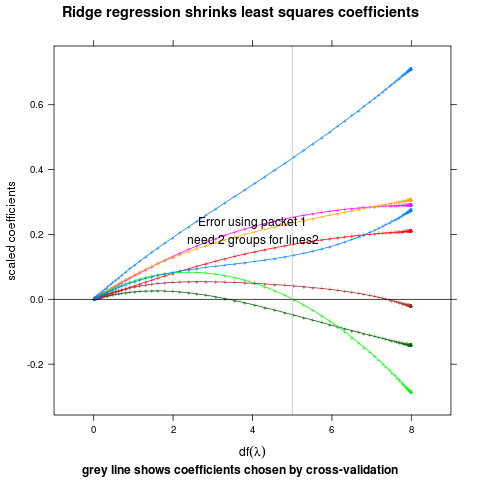Positioning Method for 2 groups of longitudinal data. One curve is on top of the other one (on average), so we label the top one at its maximal point, and the bottom one at its minimal point. Vertical justification is chosen to minimize collisions with the other line. This may not work so well for data with high variability, but then again lineplots may not be the best for these data either. ```direct.label(p,"lines2") ```Do first or last, whichever has points most spread out. ```direct.label(p,"maxvar.points") ```Label first or last points, whichever are more spread out, and use a QP solver to make sure the labels do not collide. ```direct.label(p,"maxvar.qp") ```
 Please contact Toby Dylan Hocking if you are using directlabels or have ideas to contribute, thanks! Documentation website generated from source code version 2021.2.24 (git revision bb6db07 Mon, 14 Jun 2021 22:38:45 +0530) using inlinedocs. validate